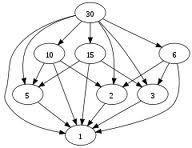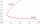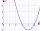# Divisibility

Is the number 761082 exactly divisible by 9? (the result is the integer and/or remainder is zero)

Result

#### Solution:

$761082 = 84564 \cdot 9 + 6$Our examples were largely sent or created by pupils and students themselves. Therefore, we would be pleased if you could send us any errors you found, spelling mistakes, or rephasing the example. Thank you!

Leave us a comment of this math problem and its solution (i.e. if it is still somewhat unclear...):Nestor
The question is ambiguous.A qualifier should be included such as "exactly as in: "Is the number 146025 exactly divisible by 6?
(all numbers are divisible by 6 only that results may not all be exact).Dr Math
In other hands - a question of divisibility is defined for integers and is defined that result is again an integer. All real numbers all divisible by six by nature, because six in non zero number.  So divisibility means that after "integer" division there is none reminder.John
call me## Next similar math problems:

1. Year 2018The product of the three positive numbers is 2018. What are the numbers?
2. DozenWhat is the product of 26 and 5? Write the answer in Arabic numeral. Add up the digits. How many of this is in a dozen? Divide #114 by this
3. IntegerFind the integer whose distance on the numerical axis from number 1 is two times smaller as the distance from number 6.
4. Collection of stampsJano, Rado, and Fero have created a collection of stamps in a ratio of 5: 6: 9. Two of them had 429 stamps together. How many stamps did their shared collection have?
5. The differenceThe difference of two numbers is 1375. If their exact quotient is 12. Find the two numbers
6. The temperatureThe temperature at 1:00 was 10 F. Between 1:00 and 2:00, the temperature dropped 15F. Between 2:00 and 3:00, the temperature rose 3F. What is the temperature at 3:00?
7. Progression12, 60, -300,1500 need next 2 numbers of pattern
8. Expression 6Evaluate expression: -6-2(4-8)-9
9. Evaluate - order of opsEvaluate the expression: 32+2[5×(24-6)]-48÷24 Pay attention to the order of operation including integers
10. Expression plus minusEvaluate expression: (-1)2 . 12 – 6 : 3 + (-3) . (-2) + 22 – (-3) . 2
11. Two integersTwo integers, a and b, have a product of 36. What is the least possible sum of a and b?
12. Evaluate 5Evaluate expression x2−7x+12x−4 when x=−1In about 12 hours in North Dakota the temperature rose from -33 degrees farenheit to 50 degrees farenheit. By how much did the temperature change?Simplify expression - which expression is equivalent to: 3(m + 2) − 4(2m − 9)Evaluate expression with negatives: (-3)+4+(-8)+(-6)+4+(-1)The temperature was 3°F and falls four degrees Fahrenheit. What is actually temperature?Solve equation with negatives: X/(-5) + 2 = -9